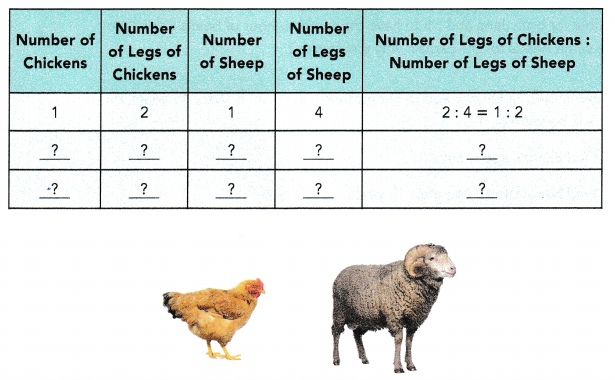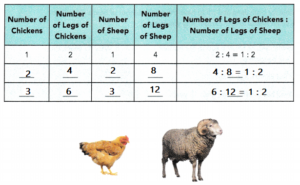# Math in Focus Grade 6 Chapter 4 Lesson 4.3 Answer Key Real-World Problems: Ratios

Go through the Math in Focus Grade 6 Workbook Answer Key Chapter 4 Lesson 4.3 Real-World Problems: Ratios to finish your assignments.

## Math in Focus Grade 6 Course 1 A Chapter 4 Lesson 4.3 Answer Key Real-World Problems: Ratios

### Math in Focus Grade 6 Chapter 4 Lesson 4.3 Guided Practice Answer Key

Solve.

Question 1.
A box contains baseball and football cards. The number of baseball cards to the number of football cards is in the ratio 5:1.
a) If the total number of cards is 1,380, find the number of each type of cards.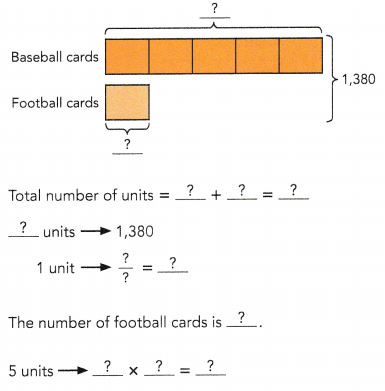The number of baseball cards is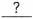.
1,150 baseball cards.
Explanation: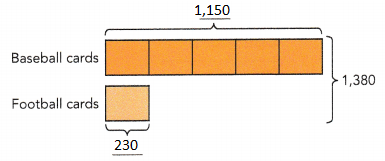Total number of units is = 5 + 1 = 6
6 units => 1,380
1 unit = 1,380/6 = 230
number of foot ball cards = 230
5 units x 230 = 1,150
The number of baseball cards is 1,150.

b) Suppose the number of baseball cards is 950, find the number of football cards.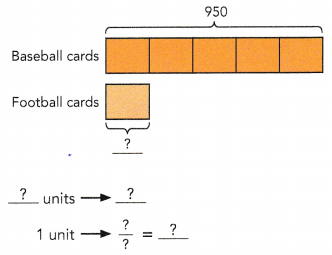The number of football cards is.
190 football cards.
Explanation: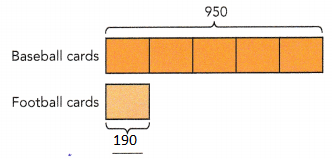5 units => 950
1 unit = 950 / 5 = 190

Question 2.
A school raised $18,000 at a charity event. The money raised was shared among three charities, A, B, and C, in the ratio 1: 2: 3. How much money did each charity receive?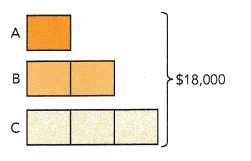Total number of units =++=units →$1 unit → $$$\frac{?}{?}$$ =$A received $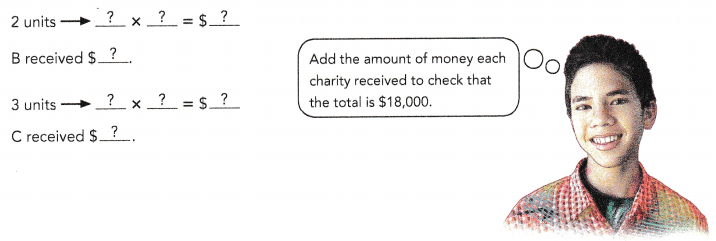Answer:$9,000
Explanation:
Total number of units = 1 +2 + 3 = 6
6 units → $18,000 1 unit →$$$\frac{18,000}{6}$$ = $3,000 A received$ 3,000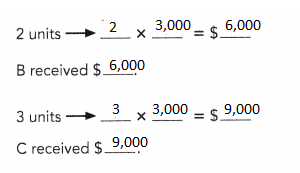Question 3.
The number of coins collected by Xavier, Yohann, and Zachary is in the ratio 2:5:8. Yohann collected 85 coins.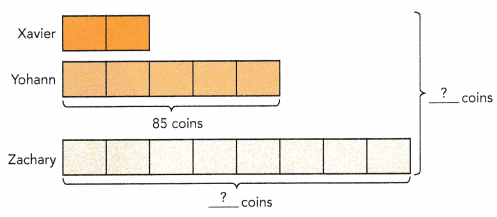a) Find the number of coins Zachary collected.
5 units →coins
1 unit → $$$\frac{?}{?}$$ =coinsunits →×=coins Zachary collectedcoins. Answer: 136 coins Explanation: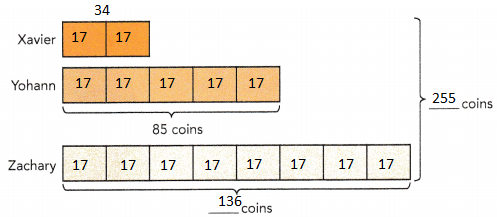the number of coins Zachary collected. 5 units → 136 coins 1 unit →$$$\frac{136}{8}$$ = 17 coins
8 units → 17 × 8 = 136 coins
Zachary collected 136 coins.

b) Find the number of coins collected by the three boys altogether.
Total number of units =++=units →×=coins
The three boys collectedcoins altogether.
255 coins
Explanation:
the number of coins collected by the three boys altogether.
Total number of units = 2 + 5 + 8
= 15
15 units → 17 ×  = 15 coins
The three boys collected 255 coins altogether.

Question 4.
At Stacey’s middle school, students either ride bikes to school, walk, or take a bus. The ratio of the number of students who ride bikes to the number who walk is 3 : 4. The ratio of the number of students who walk to the number who take a bus is 12 : 7. There are 560 students in all.
a) Find the number of students who ride bikes to school.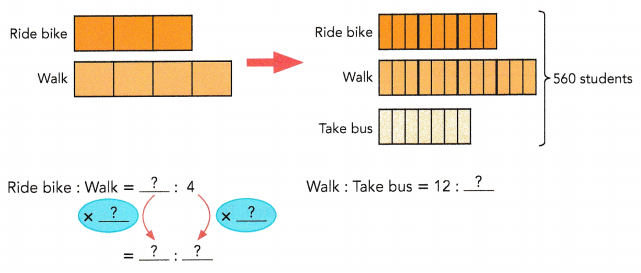So, Ride bike : Walk : Take bus =::.
Total number of units =++=units → 560 students
1 unit → $$\frac{?}{?}$$ =students
9 units →×=students
The number of students who ride bikes is.
180 bike rides
Explanation: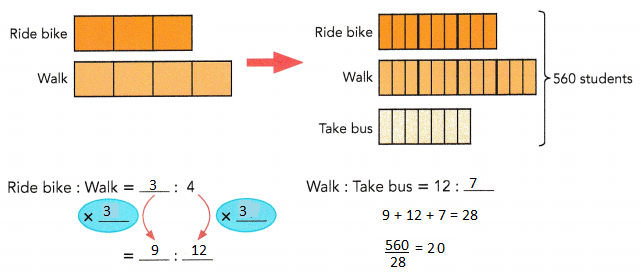Ride : Walk        Walk : bus
3:4 = 9:12              12  :  7
So, Ride bike : Walk : Take bus = 9 : 12 : 7.
Total number of units = 9 + 12 +  7 = 28
28 units → 560 students
1 unit → $$\frac{560}{28}$$ = 20 students
9 units → 9 × 20 = 180 students
The number of students who ride bikes is 180.

b) Find the number of students who walk to school.units →×=students
The number of students who walk is.
Explanation:
12 units → 12 × 20 = 240 students
The number of students who walk is 240.

c) Find the number of students who take a bus to school.units →×=students
The number of students who take a bus is.
Explanation:
7 units → 7 × 20 = 140 students
The number of students who take a bus is 140.

Question 5.
Claire keeps some green and red plates in a cabinet. The ratio of the number of green plates to the number of red plates is 2 : 1. She adds 18 more red plates in the cabinet and the ratio becomes 4 : 5.
Before
The ratio of the number of green plates to the number of red plates is:.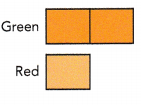After
The ratio of the number of green plates to the number of red plates becomes:.
The length of the model forplates does not change. The model forplates is divided intounits.
The model for red plates is divided into units of the same size and additional units are added to make the total lengthunits.
The additional units represent thered plates Claire adds in the cabinet.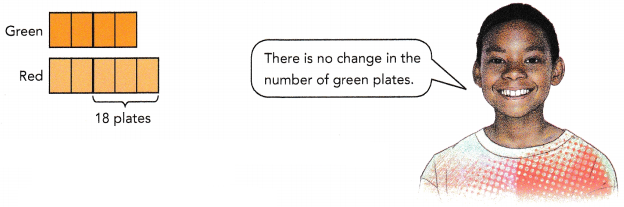Before
The ratio of the number of green plates to the number of red plates is 2 : 1.

After
The ratio of the number of green plates to the number of red plates becomes 2 : 15.
The length of the model for green plates does not change. The model for green plates is divided into 2 units.
The model for red plates is divided into units of the same size and additional units are added to make the total length 5 units.
The additional units represent the 6 red plates Claire adds in the cabinet.

a) How many green plates are there in the cabinet?
3 units →plates
1 unit → $$\frac{?}{?}$$ =platesunits →×=plates
There aregreen plates in the cabinet.
8 green plates
Explanation:
3 units → 6 plates
1 unit → $$\frac{6}{3}$$ = 2 plates
4 units → 4 × 2 =8plates
There are 8 green plates in the cabinet.

b) How many red plates are there in the cabinet in the end?units →×=plates
There arered plates in the cabinet in the end.
30 Red plates
Explanation:
5units → 5 ×6 =30 plates
There are 30 red plates in the cabinet in the end.

### Math in Focus Course 1A Practice 4.3 Answer Key

Question 1.
A rope is cut into three pieces P, Q, and R. The lengths of the pieces are in the ratio 3 : 5 : 7. If the rope is 33 feet 9 inches long, find the lengths of P, Q, and R.
P = 81in , Q = 135in, R = 189in.
Explanation:
First convert 33 feet 9 inches into inches,
There are 12 inches in 1 foot,
33 × 12 = 396.
= 396 + 9 = 405
3 : 5 : 7 = 405
3x + 5x + 7x = 405
15x = 405
x = 27
3x = 3 x 27 = 81
5x = 5 x 27 = 135
7x = 7 x 27 = 189
P = 81 , Q = 135 , R = 189.

Question 2.
Alice, Bernice, and Cheryl shared some stamps in the ratio 8 : 9 : 18. Alice received 184 stamps. Find the number of stamps that Bernice and Cheryl each received.
Bernice 207 and Cheryl 230
Explanation:
Consider the ratios in parts
Alice 8 parts = 184
184 ÷ 8 = 23
So, 1 part = 23 stamps
Bernice has 9 parts,
9 x 23 = 207 stamps
Cheryl has 10 parts,
10 x 23 = 230 stamps.

Question 3.
Alan, Gil, and Deb’s point score in a video game was in the ratio 2 : 3 : 4. Deb scored 114,400 points.
a) How many points did Gil score?
85,800 points
Explanation:
Let the points scored by Alan, Gil and Deb be
2x, 3x, and 4x
Deb scored 114,400
So, 4x = 114400
x = 28600
Gil scored 3x = 85800

b) How many points did they score in all?
257,400 points
Explanation:
Let the points scored by Alan, Gil and Deb be
2x, 3x, and 4x
Deb scored 114,400
So, 4x = 114400
x = 28600
Alan scored 2x = 2 x 28600 = 57200
Gil scored 3x = 85800
Alan scored 57200 + Gil scored 85800 + Deb scored 114400 = 257,400

Question 4.
Lara has three cats: Socks, Princess, and Luna. The ratio of Socks weight to Princess’s weight is 4 : 5. The ratio of Princess’s weight to Luna’s weight is 6 : 7. What is the ratio of Socks weight to Luna’s weight?
Ratio of Socks weight to Luna’s weight = 24 : 35
Explanation:
Let Socks weight = s
Princess weight = p
Luna’s weight = l
The ratio of Socks weight to princess weight is 4 : 5 s : p = 4 : 5
s / p = 4 / 5
The ratio of princesses weight to Luna’s weight is 6 : 7
p : l = 6 : 7
p / l = 6 / 7
the ratio of socks weight to Luna’s weight,
Multiply both given ratios (s / p) X (p / l) = (4 / 5) X (6 / 7)
s / l = 24 / 35
s : l = 24 : 35
Ratio of socks weight to Luna’s weight is 24 : 35

Question 5.
Danny poured 78 liters of water into containers X, Y, and Z. The ratio of the volume of water in Container X to the volume of water in Container Y is 5 : 2. The ratio of the volume of water in Container Y to the volume of water in Container Z is 8 : 11.
a) How much water was poured into Container X?
40 liters
Explanation:
x + y + z = 78
The ratio of the volume of water in Container X to the volume of water in Container Y is 5 : 2.
x : y = 5 : 2
The ratio of the volume of water in Container Y to the volume of water in Container Z is 8 : 11.
y : z = 8 : 11
x : y = 20 : 8 , and y : z = 8 : 11
Now, both ratios we can rewrite as one,
x : y : z = 20 : 8 : 11
20 + 8 + 11 = 39 units
Three containers contain 78 liters and that is 39 units, let’s find how many litters in 1 unit.
78 / 39 = 2 litters per unit
x = 20 x 2 = 40

b) How much more water was poured into Container X than Container Z?
18 liters
Explanation:
x + y + z = 78
The ratio of the volume of water in Container X to the volume of water in Container Y is 5 : 2.
x : y = 5 : 2
The ratio of the volume of water in Container Y to the volume of water in Container Z is 8 : 11.
y : z = 8 : 11
x : y = 20 : 8 , and y : z = 8 : 11
Now, both ratios we can rewrite as one,
x : y : z = 20 : 8 : 11
20 + 8 + 11 = 39 units
Three containers contain 78 liters and that is 39 units, let’s find how many litters in 1 unit.
78 / 39 = 2 litters per unit
x = 20 x 2 = 40
y = 8 x 2 = 16
z = 11 x 2 = 22
x – z = 40 – 22 = 18

Question 6.
The ratio of the number of mystery books to the number of science fiction books is 4 : 3. The ratio of the number of science fiction books to the number of biographies is 4 : 5. If there are 48 science fiction books, find the total number of books.
172 books
Explanation:
The ratio of the number of mystery books to the number of science fiction books is 4 : 3.
Mystery  books = 48 / 3 = 16
16 x 4 = 64
Biography books = 48 / 4 = 12
12 x 5 = 60
The ratio of the number of science fiction books to the number of biographies is 4 : 5.
If there are 48 science fiction books
The total number of books = 60 + 64 + 48 = 172

Question 7.
In a music room, the ratio of the number of clarinets to the number of flutes was 3 : 4. After the school bought another 24 flutes, the ratio became 3 : 8. How many clarinets were there in the music room?
18 clarinets
Explanation:
The ratio of the number of clarinets to the number of flutes was 3 : 4.
clarinets (c) : flutes (f) = 3 : 4
4c = 3f
After the school bought another 24 flutes, the ratio became 3 : 8.
$$\frac{c}{(f + 24)}$$ = $$\frac{3}{8}$$
Number of clarinets in music room
8=3f + 72 (3f = 4c)
8c = 4c + 72
4c = 72
c = 18

Question 8.
In a school gym, the ratio of the number of boys to the number of girls was 4 : 3. After 160 boys left the gym, the ratio became 4 : 5. How many girls were there in the gym?
300 girls
Explanation:
The ratio of the number of boys to the number of girls was 4 : 3.
b/g = 4/3
After 160 boys left the gym, the ratio became 4 : 5.
(b-160)/g = 4/5
g = 300

Question 9.
The ratio of the number of men to the number of women on a bus was 2 : 3. At a bus stop, 4 women got off and the ratio became 4 : 5.
a) How many men were on the bus?
16 men

b) How many women were on the bus in the end?
20 women
Explanation:
ratio of men to women is 2:3
2 and 3 have to be multiplied by some number, call it x, to determine the exact number of men and women
2x/3x is how to write the ratio
4 women got off the bus
2x is the number of men and 3x – 4 is the number of women
2x/(3x – 4) is the ratio now which equals 4/5
2x/(3x – 4) = 4/5
cross multiply
5(2x) = 4(3x – 4)
10x = 12x – 16
10x – 12x = – 16
-2x = -16
2x = 16
x = 16/2
x = 8
2 x 8 = 16 and 3 x 8 = 24,
so the original ratio was 16/24 which is 2/3
4 women got off the bus
16 men and 24 – 4 = 20 women

Question 10.
CD The number of chickens to the number of ducks on a farm was 6 : 5. After 63 ducks were sold, there were 3 times as many chickens as ducks left,
a) How many chickens were there on the farm?
126 chickens
Explanation:
Let there be 6x chickens and 5x ducks
Ratio of chickens and ducks = 6 : 5.
When 63 ducks are sold, by given condition,
$$\frac{6x}{(5x – 63)}$$ = $$\frac{3}{1}$$
6x = 15x – 189
9x = 189
x = $$\frac{189}{9}$$
x = 21
6x = 6 x 21 = 126
Therefore, 126 chickens were there in the farm.

b) How many chickens and ducks were there altogether on the farm in the end?
168 ducks
Explanation:
Total number of chickens and ducks in the end are
(6x) + (5x63) = 11x 63
= 11 x 21 63
= 231 63 = 168

Question 11.
The ratio of the volume of fruit juice to the volume of smoothies served at a party was 4$$\frac{1}{2}$$ : 2.4. There were 35 liters more fruit juice served than smoothies. Find the volume of smoothies served.
40 liters
Explanation:
4$$\frac{1}{2}$$ : 2.4
35 liters more fruit juice served than smoothies
4$$\frac{1}{2}$$ = $$\frac{9}{2}$$ = 4.5
4.5 : 2.4  ::  x + 35 : x
4.5 x :: 2.4 (x + 35)
4.5 x :: 2.4x + 84
4.5x – 2.4x :: 84
2.1 x = 84
x = 84/2.1
x = 40

Question 12.
A piece of ribbon is cut into two shorter pieces in the ratio 2.8 : 1.25. The difference in the length of the two shorter pieces is 80.6 centimeters. What is the length of the original piece of ribbon?
210.6cm
Explanation:
The lengths of the two pieces are in the ratio 2.8 : 1.25,
the lengths are 2.8x and 1.25x, for some positive number, x.
2.8x – 1.25x = 80.6
1.55x = 80.6
x = 52
Length of larger piece = 2.8x = 2.8 X 52 = 145.6 cm
Length of smaller piece = 1.25x = 1.25 X 52) = 65 cm
length of the original piece of ribbon = 145.6 + 65 = 210.6cm

Question 13.
Math journal The ratio of the number of beads collected by Jane to the number of beads collected by Jill is 7 : 3. Jane gave some beads to Jill. Is it possible for both Jane and Jill to have the same number of beads after Jane gave some beads to Jill? Explain why you think so.
YES
Explanation:
The ratio of the number of beads collected by Jane to the number of beads collected by Jill is 7 : 3
7x : 3x
Let ratio coefficient is = x  , So Jane have total beads  = 7 x and Jill have total beads  = 3
Now we assume that Jane gave ‘ y ‘ beads to Jill to have same number of beads for both , So
7x – y = 3x + y
7x – 3 x = y + y
4x = 2y
y = 2x
yes, it possible for both Jane and Jill to have the same number of beads after Jane gave some beads to Jill.

Question 14.
Today the ratio of Elinor’s age to her mother’s age is 3 : 8. After 15 years, the ratio will become 6 : 11.
a) Find Elinor’s age today.
15 years
Explanation:
Today the ratio of Elinor’s age to her mother’s age is 3 : 8
3x + 15 : 8x + 15 = 6 : 11
(3x + 15 ) x 11 = (8x + 15) x 6
33x + 165 = 48x + 90
48x -33x = 165 – 90
15x = 75
x = 75 / 15
x = 5

b) Find her mother’s age after 15 years.
55 years
Explanation
3x + 15 : 8x + 15 = 6 : 11
her mother’s age after 15 years.
11x = 11 x 5
= 55 years

Question 15.
The ratio of Mike’s savings to Nick’s savings was 4 : 3. After Mike saved another $120 and Nick saved another$60, the ratio became 8 : 5. What was their combined savings before each of them saved the additional money?
$210 Explanation: The ratio of Mike’s savings to Nick’s savings was 4 : 3 4 : 3 4x : 3x 4x + 120 : 3x + 60 = 8 : 5 4x + 120 = 8x 4x = 120 x = 120/4 x = 30 4 x 30 + 3 x 30 = 120+90 =$210

Brain @ Work

Question 1.
ABCD is a rectangle. BD is a straight line that cuts the rectangle into equal halves. The ratio of the area of P to the area of Q is 2 : 5, and the ratio of the area R to the area of S is 4 : 3. The area of S is 9 square centimeters.
a) Find the ratio of the area of R to the area of the rectangle.
12 square centimeter
Explanation:
The ratio of the area of P to the area of Q is 2 : 5,
the ratio of the area R to the area of S is 4 : 3. The area of S is 9 square centimeters.
P : Q :: 2 : 5
R : S :: 4 : 3
R : 9 :: 4 : 3
R = (9 x 4) / 3
R =  12 square centimeter

b) Find the area of the rectangle.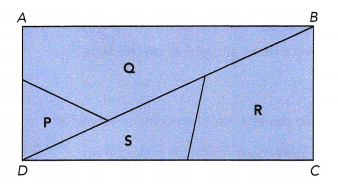42 square centimeters
Explanation:
Area of  rectangle
S+R = P + Q
9 + 12 = P + Q
P + Q = 21
Area of  rectangle
S+R + P + Q
21 + 21 = 42 square centimeters

Question 2.
A farmer raises chickens and sheep on his farm. The ratio of the total number of legs of the chickens to the total number of legs of the sheep is 4 : 7. Find the minimum number of chickens and sheep on his farm. Copy and complete the table to solve the problem. (Hint: Make a list and solve the problem using guess and check.)